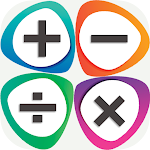1K+

# Math Learner : Math Puzzles APK

Version: 1.6 code 7

Developer: alphaGbrand studio

Released: 2021-04-27

Updated: 2022-07-29## The description of Math Learner : Math Puzzles APK

Learning mathematics has become one of the priorities of our current era because of its contributions in various fields, including but not limited to its role in physics and space engineering, as well as in its use in our daily and school
The mathematics learning application helps you learn quickly and in very simple with easy ways, as you can use the application at home
Math learn puzzles enables you to learn addition, subtraction, multiplication and division operations. The application enables you to solve Algebra Equation and Quadratic Equation
The application is suitable for teachers as well, because math brain puzzles app helps the teacher to create tests for students and export the test file and share it with the students
How to use the mathematics learning app in my school and when you riding the subway or car
- Addition: we add the spaces of the two numbers from zero
- Subtraction : converting subtraction into addition with changing the sign of the second number
- Multiplication: multiply two numbers, taking into account the sign
- Division: Keeping the first number and converting the division into multiplication and reciprocal of the second number
How to solve equations in my school:
- To solve Algebra Equation, it is by determining the value of the unknown number, and you can change the place of the number from one end to the other, provided that its sign is changed
- To solve this equation there are two methods, the first is by calculating the Discriminant, and the second method is by factorize the equation and converting it into two equations of the first degree and following the same steps mentioned above.
How to calculate fractional numbers: We unify the denominator between the two mixed numbers and then we add the numerators
With Math brain puzzles app you can easily learn how to calculate percentage and calculate the power of a root number
Features of the application of learning mathematics:
- Supporting the four operations such as multiplication, division, addition and subtraction
- The application supports Arabic, French, Spanish, English, Malay and the Russian language, and in the near future we will support more languages
- Calculate percentages and the square of a natural integer
- solve math Equation and Quadratic Equation with one button click
Share the application of learning mathematics with your friends to spread the benefit and contribute to building an advanced scientific community.

Update on
2022-07-29
Package name
com.alphaGbrand.mathlearnpuzzles
Version
1.6
Installs
1.000++
File size
13.035.084 bytes
Requirements
Android 5.0+
Content rating
Rated for 0+

### All versions(3)

We use cookies and other technologies on this website to enhance your user experience.